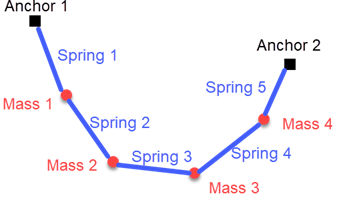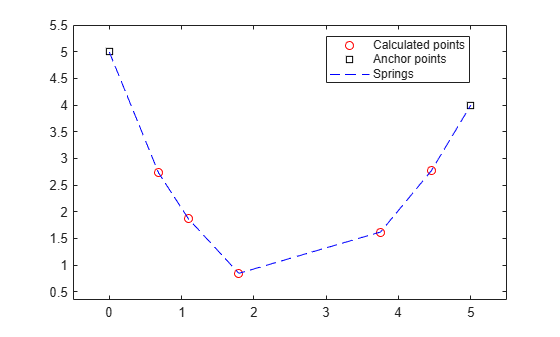Minimize Energy of Piecewise Linear Mass-Spring System Using Cone Programming, Solver-Based

This example shows how to find the equilibrium position of a mass-spring system hanging from two anchor points. The springs have piecewise linear tensile forces. The system consists of $n$ masses in two dimensions. Mass $i$ is connected to springs $i$ and $i+1$. Springs $1$ and $n+1$ are also connected to separate anchor points. In this case, the zero-force length of spring $i$ is a positive length $l\left(i\right)$, and the spring generates force $k\left(i\right)q$ when stretched to length $q+l\left(i\right)$. The problem is to find the minimum potential energy configuration of the masses, where potential energy comes from the force of gravity and from the stretching of the nonlinear springs. The equilibrium occurs at the minimum energy configuration.

This illustration shows five springs and four masses suspended from two anchor points.The potential energy of a mass $m$ at height $h$ is $mgh$, where $g$ is the gravitational constant on Earth. Also, the potential energy of an ideal linear spring with spring constant $k$ stretched to length $q$ is $k{q}^{2}/2$. The current model is that the spring is not ideal, but has a nonzero resting length $l$.

The mathematical basis of this example comes from Lobo, Vandenberg, Boyd, and Lebret . For a problem-based version of this example, see Minimize Energy of Piecewise Linear Mass-Spring System Using Cone Programming, Problem-Based.

Mathematical Formulation

The location of mass $i$ is $x\left(i\right)$, with horizontal coordinate ${x}_{1}\left(i\right)$ and vertical coordinate ${x}_{2}\left(i\right)$. Mass $i$ has potential energy due to gravity of $gm\left(i\right){x}_{2}\left(i\right)$. The potential energy in spring $i$ is $k\left(i\right)\left(d\left(i\right)-l\left(i\right){\right)}^{2}/2$, where $d\left(i\right)$ is the length of the spring between mass $i$ and mass $i-1$. Take anchor point 1 as the position of mass 0, and anchor point 2 as the position of mass $n+1$. The preceding energy calculation shows that the potential energy of spring $i$ is

$Energy\left(i\right)=\frac{k\left(i\right)\left(‖x\left(i\right)-x\left(i-1\right)‖-l\left(i\right){\right)}^{2}}{2}$.

Reformulating this potential energy problem as a second-order cone problem requires the introduction of some new variables, as described in Lobo . Create variables $t\left(i\right)$ equal to the square root of the term $Energy\left(i\right)$.

$t\left(i\right)=\sqrt{\frac{k\left(i\right)\left(‖x\left(i\right)-x\left(i-1\right)‖-l\left(i\right){\right)}^{2}}{2}}.$

Let $e$ be the unit column vector $\left[\genfrac{}{}{0}{}{0}{1}\right]$. Then ${x}_{2}\left(i\right)={e}^{T}x\left(i\right)$. The problem becomes

$\underset{x,t}{\mathrm{min}}\left(\sum _{i}gm\left(i\right){e}^{T}x\left(i\right)+‖t{‖}^{2}\right).$               (1)

Now consider $t$ as a free vector variable, not given by the previous equation for $t\left(i\right)$. Incorporate the relationship between $x\left(i\right)$ and $t\left(i\right)$ in the new set of cone constraints

$‖x\left(i\right)-x\left(i-1\right)‖-l\left(i\right)\le \sqrt{\frac{2}{k\left(i\right)}}t\left(i\right).$   (2)

The objective function is not yet linear in its variables, as required for coneprog. Introduce a new scalar variable $y$. Notice that the inequality $‖t{‖}^{2}\le y$ is equivalent to the inequality

$‖\left[\genfrac{}{}{0}{}{2t}{1-y}\right]‖\le 1+y$.                               (3)

Now the problem is to minimize

$\underset{x,t,y}{\mathrm{min}}\left(\sum _{i}gm\left(i\right){e}^{T}x\left(i\right)+y\right)$                     (4)

subject to the cone constraints on $x\left(i\right)$ and $t\left(i\right)$ listed in (2) and the additional cone constraint (3). Cone constraint (3) ensures that $‖t{‖}^{2}\le y$. Therefore, problem (4) is equivalent to problem (1).

The objective function and cone constraints in problem (4) are suitable for solution with coneprog.

MATLAB® Formulation

Define six spring constants $k$, six length constants $l$, and five masses $m$.

k = 40*(1:6);
l = [1 1/2 1 2 1 1/2];
m = [2 1 3 2 1];

Define the approximate gravitational constant on Earth $g$.

g = 9.807;

The variables for optimization are the ten components of the $x$ vectors, the six components of the $t$ vector, and the $y$ variable. Let v be the vector containing all these variables.

• [v(1),v(2)] corresponds to the 2-D variable $x\left(1\right)$.

• [v(3),v(4)] corresponds to the 2-D variable $x\left(2\right)$.

• [v(5),v(6)] corresponds to the 2-D variable $x\left(3\right)$.

• [v(7),v(8)] corresponds to the 2-D variable $x\left(4\right)$.

• [v(9),v(10)] corresponds to the 2-D variable $x\left(5\right)$.

• [v(11):v(16)] corresponds to the 6-D vector $t$.

• v(17) corresponds to the scalar variable $y$.

Using these variables, create the corresponding objective function vector f.

f = zeros(size(m));
f = [f;g*m];
f = f(:);
f = [f;zeros(length(k)+1,1)];
f(end) = 1;

Create the cone constraints corresponding to the springs between the masses (2)

$‖x\left(i\right)-x\left(i-1\right)‖-l\left(i\right)\le \sqrt{\frac{2}{k\left(i\right)}}t\left(i\right)$.

The coneprog solver uses cone constraints for a variable vector $v$ in the form

$‖{A}_{sc}\cdot v-{b}_{sc}‖\le {d}_{sc}^{T}v-\gamma$.

In the following code, the Asc matrix represents the term $‖x\left(i\right)-x\left(i-1\right)‖$, with bsc = [0;0]. The cone variable dsc = $\sqrt{2/k\left(i\right)}$ and the corresponding gamma = $-l\left(i\right).$

d = zeros(1,length(f));
Asc = d;
Asc([1 3]) = [1 -1];
A2 = circshift(Asc,1);
Asc = [Asc;A2];
ml = length(m);
dbase = 2*ml;
bsc = [0;0];
for i = 2:ml
gamma = -l(i);
dsc = d;
dsc(dbase + i) = sqrt(2/k(i));
conecons(i) = secondordercone(Asc,bsc,dsc,gamma);
Asc = circshift(Asc,2,2);
end

Create the cone constraints corresponding to the springs between the end masses and the anchor points by using the anchor points for the locations of the end masses, as in the preceding code.

x0 = [0;5];
xn = [5;4];

Asc = zeros(size(Asc));
Asc(1,(dbase-1)) = 1;
Asc(2,dbase) = 1;
bsc = xn;
gamma = -l(ml);
dsc = d;
dsc(dbase + ml) = sqrt(2/k(ml));
conecons(ml + 1) = secondordercone(Asc,bsc,dsc,gamma);

Asc = zeros(size(Asc));
Asc(1,1) = 1;
Asc(2,2) = 1;
bsc = x0;
gamma = -l(1);
dsc = d;
dsc(dbase + 1) = sqrt(2/k(1));
conecons(1) = secondordercone(Asc,bsc,dsc,gamma);

Create the cone constraint (3) corresponding to the $y$ variable

$‖\left[\genfrac{}{}{0}{}{2t}{1-y}\right]‖\le 1+y$

by creating the matrix Asc which, when multiplied by the v vector, gives the vector $\left[\genfrac{}{}{0}{}{2t}{-y}\right]$. The bsc vector corresponds to the constant 1 in the term $1-y$. The dsc vector, when multiplied by v, returns $y$. And gamma = $-1$.

Asc = 2*eye(length(f));
Asc(1:dbase,:) = [];
Asc(end,end) = -1;
bsc = zeros(size(Asc,1),1);
bsc(end) = -1;
dsc = d;
dsc(end) = 1;
gamma = -1;
conecons(ml+2) = secondordercone(Asc,bsc,dsc,gamma);

Finally, create lower bounds corresponding to the $t$ and $y$ variables.

lb = -inf(size(f));
lb(dbase+1:end) = 0;

Solve Problem and Plot Solution

The problem formulation is complete. Solve the problem by calling coneprog.

[v,fval,exitflag,output] = coneprog(f,conecons,[],[],[],[],lb);
Optimal solution found.

Plot the solution points and the anchors.

pp = v(1:2*ml);
pp = reshape(pp,2,[]);
pp = pp';
plot(pp(:,1),pp(:,2),'ro')
hold on
xx = [x0,xn]';
plot(xx(:,1),xx(:,2),'ks')
xlim([x0(1)-0.5,xn(1)+0.5])
ylim([min(pp(:,2))-0.5,max(x0(2),xn(2))+0.5])
xxx = [x0';pp;xn'];
plot(xxx(:,1),xxx(:,2),'b--')
legend('Calculated points','Anchor points','Springs','Location',"best")
hold offYou can change the values of the parameters m, l, and k to see how they affect the solution. You can also change the number of masses; the code takes the number of masses from the data you supply.

References

 Lobo, Miguel Sousa, Lieven Vandenberghe, Stephen Boyd, and Hervé Lebret. “Applications of Second-Order Cone Programming.” Linear Algebra and Its Applications 284, no. 1–3 (November 1998): 193–228. https://doi.org/10.1016/S0024-3795(98)10032-0.